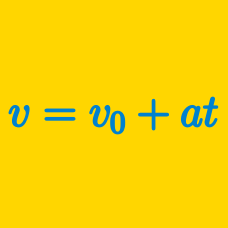Classical Mechanics

# Position, Velocity, and AccelerationSuppose that the displacement $s$ of an aeroplane can be expressed as a function of time $t$ in the following way: $s(t) = 8 t^2 + 10 t .$ What is the magnitude of the acceleration of the object?

Point $P$ moves along the $x$-axis in such a way that its position is $x(t)=6-2t,$ where $t$ denotes elapsed time. If $t$ has changed from $0$ to $12,$ what is the average velocity of point $P?$

At 5:30 pm, Stacey was driving her car at a speed of $30\text{ mph}$ on a straight avenue. Suddenly she notices that she had forgotten to bring her purse, so she immediately makes a U-turn and heads back to her house. Now she looks at her watch, which says 6:00 pm. If her current speed is $40\text{ mph},$ what is the magnitude of her average acceleration from 5:30 to 6:00 pm?

John is jogging from his home to the park, and back. If the distance between his home and the park is $700$ yards and John has jogged for a total of $14$ minutes, what is his average velocity, $\vec{v}$?

I'm driving along at a constant speed in my 3-meter-long car when I notice that I am accidentally driving through a red light. If the rear end of my car passes under the light 0.3 seconds after the front end of my car, how fast am I driving in m/s?

×